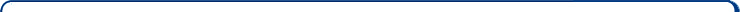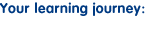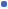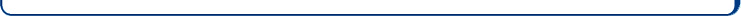Home > ICT showme > ExcelWhat is a spreadsheet?Add, subtract, divide and multiplyDeterming totalsCounting itemsDetermining the average of a listDetermining the largest amount in a listDetermining the smallest amount in a listCalculating a percentageCalculating the percentage of an amountSetting the printing rangeFormatting cellsPassword and protectionUsing multiple worksheets at the same timeEnter/edit data on worksheetsDrag and drop and AutofillCopy and CutCreating graphs